## 8  Comprehension

Now we develop a λ-calculus for S, just as Section A 8 did for SS.

The new calculus is based on a type-theoretic presentation [Tay99, Section 2.2] of Zermelo’s original set theory, with ΣX playing the role of the powerset (℘ X) and the object {XE} from Section 4 that of comprehension. Whereas comprehension is often presented in a way that allows each term to belong to many types, the new operators admit, i and I in our calculus, whilst perhaps being a little bureaucratic, ensure that each term has a unique type.

Writing D for the category obtained from the new calculus as explained in [Tay99, Sections 4.3 & 4.7] and more briefly in Remark A 2.7, we shall show in the following sections that SD. This will be done by means of a weak normalisation theorem, thereby showing that we have stated enough equations. It is a categorical equivalence, rather than the isomorphism that we had for SS, because we’re now manipulating types.

Remark 8.1 In the calculus that we have in mind for set theory, the rules for the powerset ℘ X≡ΩX are those of the restricted λ-calculus, where ξ[a] means a∈{X ∣ ξ}. Then comprehension has formation and introduction rules,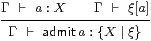elimination rules,

 x:{X ∣ ξ}   ⊢   iX,ξ x:X           x:{X ∣ ξ}   ⊢   ξ[iX,ξ x]

and β and η-rules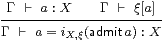x:{X ∣ ξ}   ⊢   x=admit( iX,ξ x).

The intent of these rules is to specify when there is a term admita that makes the triangle commute: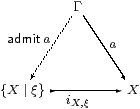Notice that we have required ξ:℘ X to be a closed term (defined in the empty context), because we do not want to get involved in dependent types in this paper.

Remark 8.2 The rules that we give below for our own monadic situation are not very pretty if seen as a piece of pure λ-calculus. But they are in the spirit of the treatment of comprehension above, so long as we read the the type-formation rule as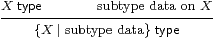where any predicate ξ:℘ X provides subtype data in set theory, and the nucleus E (Definition 4.3) does so for our own calculus. Similarly, the introduction rule meanswhere our admissibility condition is unfortunately more complicated and less intuitive than that in set theory.

You may think of

 {X ∣ E}    as     {x:X ∣ ∀φ:ΣX.Eφ x ⇔ φ x},

but this can only be internalised if ΣX is compact (so ∀φ:ΣX is meaningful) and Σ is discrete (so ⇔ is internal). These conditions (along with what is needed in Example 2.6(a) to define IX,Eθ in terms of ∃) would make S into a topos (Theorem C 11.12), and thereby bring the comprehension calculus back to that for Zermelo type theory.

Remark 8.3 As we have learned throughout this paper, we need to be explicit about the subspace topology. The elimination rule for elements ({}E0) below provides the restriction of an open subset φ of X to the subspace {XE}, which we may re-interpret as the introduction rule for predicates,For the corresponding elimination rule, we need a new operator IX,E to expand an open subset θ of the subspace to the whole space.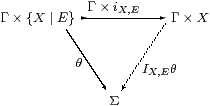For reference, we repeat Definition A 2.1,A 8.1,A 8.2 and 4.3.

Definition 8.4 The restricted λ-calculus has just the type-formation rules

 1 type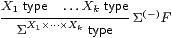but with the normal rules for λ-abstraction and application,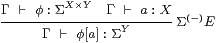together with the usual α, β and η rules.

Definition 8.5 Γ⊢ PΣX is prime if  Γ,  F3 XF P   ⇔   Px.F(λφ.φ x)).

Definition 8.6 The sober λ-calculus has the additional rules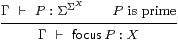focusIfocusβ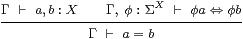T0

Definition 8.7 φ:ΣYEφ:ΣY is called a nucleus if

 F:Σ3 Y   ⊢    E(λ y.F(λφ.Eφ y))   =  E(λ y.F(λφ.φ y)).

Definition 8.8 The {}-rules of the monadic λ-calculus define the subspace itself.⎧ ⎨ ⎩ ⎫ ⎬ ⎭
F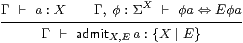⎧ ⎨ ⎩ ⎫ ⎬ ⎭
I

x:{X ∣ E}   ⊢   iX,E x:X
 ⎧ ⎨ ⎩ ⎫ ⎬ ⎭
E0
x:{X ∣ E},  φ:ΣX   ⊢ φ(iX,E x) ⇔ Eφ(iX,E x)
 ⎧ ⎨ ⎩ ⎫ ⎬ ⎭
E1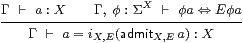⎧ ⎨ ⎩ ⎫ ⎬ ⎭
β

x:{X ∣ E}   ⊢   x=admitX,E(iX,E x)
 ⎧ ⎨ ⎩ ⎫ ⎬ ⎭
η

Definition 8.9 The Σ{}-rules say that it has the subspace topology, where IX,E expands open subsets of the subspace to the whole space.

θ:Σ{X ∣ E} ⊢   IX,Eθ:ΣX          Σ
 ⎧ ⎨ ⎩ ⎫ ⎬ ⎭

E

The β-rule says that the composite ΣX↠Σ{XE}↣ΣX is E:

φ:ΣX   ⊢   IX,E(λ x:{X ∣ E}.φ(iX,E x)) = Eφ          Σ
 ⎧ ⎨ ⎩ ⎫ ⎬ ⎭

β

Notice that this is the only rule that introduces E into the λ-expressions. The η-rule, which we first saw in Remark 2.8, says that the other composite Σ{XE}↣ΣX↠Σ{XE} is the identity:

θ:Σ{X ∣ E},  x:{X ∣ E}   ⊢   θ x ⇔ IX,Eθ(iX,E x)           Σ
 ⎧ ⎨ ⎩ ⎫ ⎬ ⎭

η.

In any type theory where terms are embedded in the definitions of types, we must check that, when two terms are equal according to the rules of the calculus, they give rise to interchangeable types. This result has the flavour of the normalisation proof that follows (Proposition 9.11), whilst being a lot simpler, so you may find it helpful to fill in the details of the verifications as a preparatory exercise.

Lemma 8.10 If E1=E2 then there is an isomorphism {XE1}≅{XE2} that commutes with the structure (admit, i and I).

Proof    The isomorphism for values is x1admit2(i1 x1) and vice versa. This is well formed, i.e. the side condition for {}I is satisfied and justifies the use of admit2, because of the equation E1=E2. The isomorphism for properties is θ1↦Σi2(I1θ1). The equations for the isomorphisms and commutativity with the structure follow easily from the β- and η-rules for the two sides.         ▫

Before embarking on the main normalisation theorem, we investigate some of the interactions between the new admit operation and the underlying sober and recursive structures. The first result is the symbolic analogue of Remark 4.11, showing that admit does the same for a general Σ-split inclusion as focus does for η.

Lemma 8.11 The standard resolution defines an isomorphism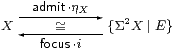where E≡ηΣX·ΣηX.

Proof    The term x:Xadmit(λφ.φ x) is well formed because of the unit law. The right hand side of Definition 8.5 is EF P, so the subspace {Σ2 XE} exactly captures the primes and

 P:{Σ2 X ∣ E}   ⊢   focus(i P):X

is well formed. Then focus(i(admit(λφ.φ x)))  =  focus(λφ.φ x)  =  x by {}β and focusη. Conversely, admitX(focus(i P)))  =  admit(i P)  =  P by focusβ and {}η.         ▫

The interaction between admit and substitution, i.e. cut elimination, is as expected, cfLemma A 8.4.

Lemma 8.12 Let Γ⊢admitX,E a:{XE} be a well formed term and u:Δ→Γ a substitution [Tay99, Section 4.3]. Then Δ⊢admitX,E u*a:{XE} is also well formed, and

Proof    In the context [Δ, φ:ΣX], since E and φ do not depend on Γ,

 φ(u* a)  ≡  u*(φ a)   ⇔   u*(Eφ a)  ≡  Eφ(u*a).

This is consistent with the {}β- and η-rules when we put u*(iX,Ea) ⇔ iX,E(u*a), and with the Σ{}-rules since u*(IX,Eθ) ⇔ IX,E(u*θ).         ▫

Much of the business of Section A 8, which introduced the focus operation to handle sobriety, was to eliminate it. In particular, Proposition A 8.10 showed that focus is redundant for logical terms (i.e. of type ΣU), whilst Section A 910 replaced it with descriptions for numerical terms (i.e. of type ℕ). The following result adds a new case to that reduction, for terms of type {YE}, so focus can still be pushed to the outside of any term of the comprehension calculus, or eliminated altogether.

Lemma 8.13 If Γ⊢ P2 {YE} is prime then

 focus{Y ∣ E} P  =  admitY,E(focusY(Σ2 iY,E P)).

So {YE} is sober, cf. Lemma 6.9.

Proof    Since Γ⊢Σ2 i P2 Y is also prime (Lemma A 4.6), we may form

 Γ  ⊢   a ≡ focusY(Σ2 i P):Y,

which satisfies

 φ a ⇔ φ(focusY(Σ2 i P)) ⇔ Σ2 i P φ     focusβ ⇔ P(Σiφ)   ⇔   P(λ y.φ(i y))     definition of Σ2 i ⇔ P(λ y.E φ (i y))     {}E1 ⇔ E φ a     in the same way,

so we may then form Γ⊢admitY,E(focusY2 i P)). Applying θ:Σ{XE},

 θ(admit(focusY(Σ2 i P))) ⇔ Iθ(focusY(Σ2 i P))     {}β, Σ{}η ⇔ Σ2 i P(Iθ)     focusβ ⇔ P(Σi(Iθ))    ⇔   Pθ      Σ{}η ⇔ θ(focus{Y ∣ E} P)     focusβ,

whence the result follows by T0.         ▫

Finally, admit can also be moved to the outside of the term, although that is in fact the normalisation theorem to which the next two sections are devoted. Here we just consider the interaction with recursion, which is the symbolic analogue of Proposition 6.14.

[This proof requires equational hypotheses (Remark E 2.7).]

Lemma 8.14 Let X={YE} with terms

 Γ  ⊢   n:ℕ,      Γ  ⊢   z:Y     and     Γ,  m:ℕ,  y:Y   ⊢   s(m,y):Y

such that Γ⊢admitz:X and Γ,  m:ℕ,  x:Xadmits(m,i x):X are well formed. Then